# 拖曳声呐流噪声场模型研究Research on Towed Sound Turbulence Noise Field Model

DOI: 10.12677/OJAV.2019.73010, PDF, HTML, XML, 下载: 373  浏览: 720  国家自然科学基金支持

Abstract: With the widespread application of towed hydrophones on marine detection and underwater vehicles, the performance requirements of hydrophone translating efficiency are increasing, and the influence of flow noise is huge, which limits its positioning detection capability. In this paper, the wave-frequency spectrum analysis method is used to give the power spectrum expression and time-space correlation function of the internal noise field of the towed line array, and the existing models are compared by numerical calculation method. The Carpenter model is simulated, improved and parameter modified, and effective noise suppression measures are obtained. The effectiveness of the flow noise model is verified by measured data.

1. 引言

2. 水听器流噪声场理论分析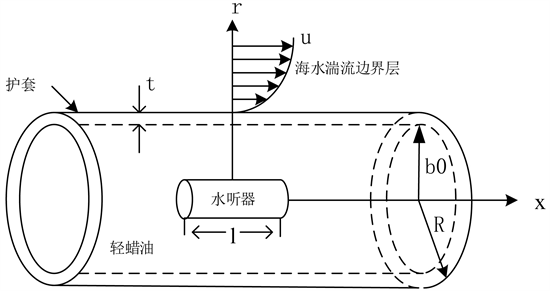Figure 1. Subdivision diagram of common horizontal towed line array sonar

$p\left(z,a,t\right)=\underset{-\infty }{\overset{\infty }{\int \text{ }\int }}\text{ }\text{ }s\left({k}_{x},\omega \right){\text{e}}^{i\left({k}_{x}x-\omega t\right)}\text{d}{k}_{x}\text{d}\omega$ (1)

$G\left(x,r,t\right)=\underset{-\infty }{\overset{\infty }{\int }}\text{ }\text{ }s\left({k}_{x},\omega \right)h\left({k}_{x},r,\omega \right){\text{e}}^{i\left({k}_{x}x-\omega t\right)}\text{d}{k}_{x}\text{d}\omega$ (2)

$\begin{array}{c}R\left(L,{r}_{1},{r}_{2},\tau \right)=G\left(x,{r}_{1},t\right){G}^{*}\left(x-L,{r}_{2},t-\tau \right)\\ =\underset{-\infty }{\overset{\infty }{\int \text{ }\int }}\text{ }\text{ }{\varphi }_{0}\left({k}_{x},\omega \right)h\left({k}_{x},{r}_{1},\omega \right){h}^{*}\left({k}_{x},{r}_{2},\omega \right){\text{e}}^{i{k}_{x}L}{\text{e}}^{-i\omega \tau }\text{d}{k}_{x}\text{d}\omega \end{array}$ (3)

$\varphi \left(L,{r}_{1},{r}_{2},\omega \right)=\underset{-\infty }{\overset{\infty }{\int }}\text{ }\text{ }{\varphi }_{0}\left({k}_{x},\omega \right)h\left({k}_{x},{r}_{1},\omega \right){h}^{*}\left({k}_{x},{r}_{2},\omega \right){\text{e}}^{i{k}_{x}L}\text{d}{k}_{x}$ (4)

${r}_{1}={r}_{2}=r$$L=0$ 的时候，得到随机压力场的自功率谱 $\varphi \left(0,r,\omega \right)$ 的表达式为：

$\varphi \left(0,r,\omega \right)=\underset{-\infty }{\overset{\infty }{\int }}\text{ }\text{ }{\varphi }_{0}\left({k}_{x},\omega \right){|h\left({k}_{x},{r}_{1},\omega \right)|}^{2}\text{d}{k}_{x}$ (5)

${\varphi }_{0}\left({k}_{x},\omega \right)=\frac{{c}^{2}{\rho }_{2}^{2}{v}_{\ast }^{3}{R}^{2}\left[{\left({k}_{x}R\right)}^{2}+1/12\right]}{{\left[\frac{{\left(\omega R-{U}_{c}{k}_{x}R\right)}^{2}}{{\left(h{v}_{*}\right)}^{2}}+{\left({k}_{x}R\right)}^{2}+1/{b}^{2}\right]}^{2.5}}$ (6)Table 1. Significance and value of Carpenter model parameters

$T\left({k}_{x},\omega \right)=\frac{{k}_{b}^{2}}{{k}_{b}^{2}-{k}_{x}^{2}}$ (7)

3. 拖曳声呐水听器阵列流噪声仿真分析

$\begin{array}{c}\stackrel{¯}{G\left(x,{r}_{0},t\right)}=\frac{1}{l}\underset{x-l/2}{\overset{x+l/2}{\int }}G\left(x,{r}_{0},t\right)\text{d}x=\frac{1}{l}\underset{x-l/2}{\overset{x+l/2}{\int }}\underset{-\infty }{\overset{\infty }{\int \text{ }\int }}\text{ }\text{ }S\left({k}_{x},\omega \right){h}_{p}\left({k}_{x},{r}_{0,}\omega \right)\text{d}{k}_{x}\text{d}\omega \text{d}x\\ =\underset{-\infty }{\overset{\infty }{\int \text{ }\int }}\text{ }\text{ }S\left({k}_{x},\omega \right){h}_{p}\left({k}_{x},{r}_{0,}\omega \right){a}_{p}\left({k}_{x}\right){\text{e}}^{i\left({k}_{x}x-\omega t\right)}\text{d}{k}_{x}\text{d}\omega \\ =\underset{-\infty }{\overset{\infty }{\int \text{ }\int }}\text{ }\text{ }S\left({k}_{x},\omega \right){h}_{p}\left({k}_{x},{r}_{0,}\omega \right){a}_{p}\left({k}_{x}\right){\text{e}}^{i\left({k}_{x}x-\omega t\right)}\text{d}{k}_{x}\text{d}\omega \\ =\underset{-\infty }{\overset{\infty }{\int \text{ }\int }}\text{ }\text{ }S\left({k}_{x},\omega \right){h}_{p}\left({k}_{x},{r}_{0,}\omega \right){a}_{p}\left({k}_{x}\right){\text{e}}^{i\left({k}_{x}x-\omega t\right)}\text{d}{k}_{x}\text{d}\omega \end{array}$ (8)

${a}_{p}\left({k}_{x}\right)=\frac{\text{sin}\left({k}_{x}l/2\right)}{{k}_{x}l/2}$ (9)

${\varphi }_{p}\left({r}_{0},\omega \right)=\underset{-\infty }{\overset{\infty }{\int }}\text{ }{\varphi }_{s}\left({k}_{x},\omega \right){|{h}_{p}\left({k}_{x},{r}_{0},\omega \right)|}^{2}{|{a}_{p}\left({k}_{x}\right)|}^{2}\text{d}{k}_{x}$ (10)

${\varphi }_{x}\left({r}_{0},\omega \right)=\underset{-\infty }{\overset{\infty }{\int }}\text{ }\text{ }{\varphi }_{0}\left({k}_{x},\omega \right){|{h}_{p}\left({k}_{x},{r}_{0},\omega \right)|}^{2}{|\frac{{J}_{0}\left({k}_{1}{r}_{0}\right)}{{J}_{0}\left({k}_{1}b\right)}|}^{2}{|{a}_{x}\left({k}_{x}\right)|}^{2}\text{d}{k}_{x}$ (11)

${a}_{x}\left({k}_{x}\right)=\text{cos}\left(\frac{{k}_{x}l}{2}\right)$ (12)

3.1. 声呐拖曳速度对流噪声的影响Figure 2. Relation between flow noise sound pressure power spectrum and towed velocity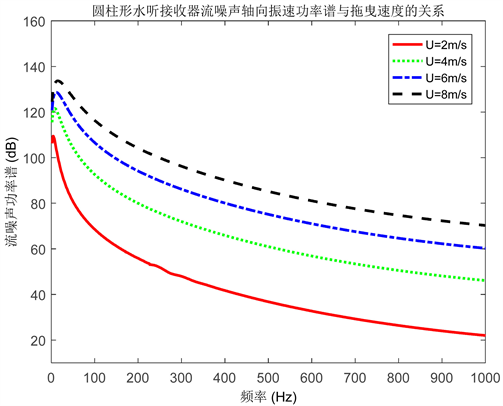Figure 3. Relation between flow noise axial vibration velocity power spectrum and towed velocity

3.2. 水听器尺度对流噪声的影响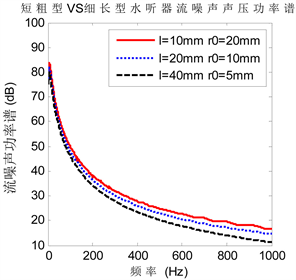Figure 4. Sound pressure power spectrum of flow noise of hydrophone with different scalesFigure 5. Axial velocity power spectrum of flow noise of hydrophones with different scales

3.3. 小结Table 2. Flow noise response under different parameters

4. 基于声学浮标的Carpenter压力模型实测验证

1) 水听器一定的时间后浮标在垂直方向上的自身扰动运动会消失。

2) 声学浮标流噪声功率谱模型相比于前文研究的拖曳线列阵模型来讲更加简化。

3) 不考虑护套传递函数和径向传播约束，即只需要考虑TBL压力起伏模型和水听器的响应函数。

${\varphi }_{0}\left(\omega \right)=\underset{-\infty }{\overset{\infty }{\int }}\text{ }\text{ }{\varphi }_{0}\left({k}_{x},\omega \right){|{a}_{p}\left({k}_{x}\right)|}^{2}\text{d}{k}_{x}$ (13)

${\varphi }_{0}\left({k}_{x},\omega \right)=\frac{{c}^{2}{\rho }_{2}^{2}{v}_{\ast }^{3}{R}^{2}\left[{\left({k}_{x}R\right)}^{2}+1/12\right]}{{\left[\frac{{\left(\omega R-{U}_{c}{k}_{x}R\right)}^{2}}{{\left(h{v}_{*}\right)}^{2}}+{\left({k}_{x}R\right)}^{2}+1/{b}^{2}\right]}^{2.5}}$ (14)

${a}_{p}\left({k}_{x}\right)=\frac{\text{sin}\left({k}_{x}l/2\right)}{{k}_{x}l/2}$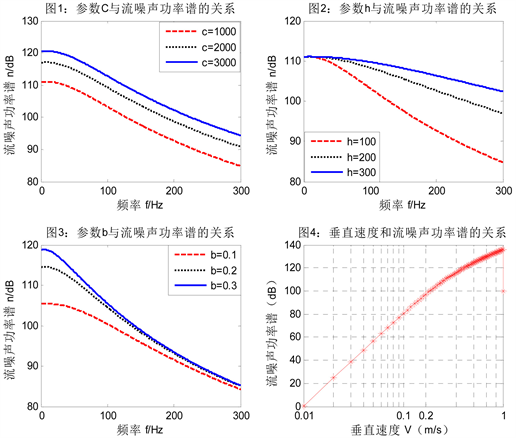Figure 6. Power spectrum of flow noise under different parameters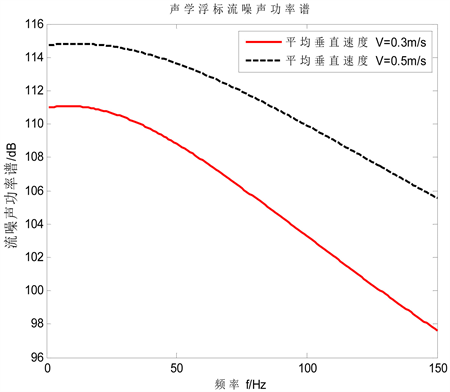Figure 7. Acoustic buoy current noise power spectrum

$R\left(L,\tau \right)=\underset{-\infty }{\overset{\infty }{\int \text{ }\int }}\text{ }\text{ }{\varphi }_{0}\left({k}_{x},\omega \right)h\left({k}_{x},{r}_{1},\omega \right){h}^{*}\left({k}_{x},{r}_{2},\omega \right){\text{e}}^{i\left({k}_{x}L-\omega \tau \right)}\text{d}{k}_{x}\text{d}\omega$ (15)

$R\left(L,0\right)\approx \underset{-\infty }{\overset{\infty }{\int }}\text{ }\text{ }{\varphi }_{0}\left({k}_{x},\omega \right){|H\left({k}_{x}\right)|}^{2}{\text{e}}^{i{k}_{x}L}\text{d}{k}_{x}$ (16)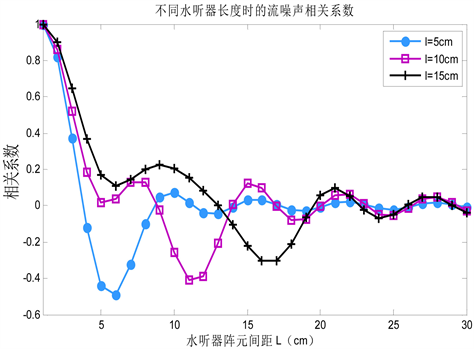Figure 8. Correlation coefficients of flow noise at different hydrophone lengths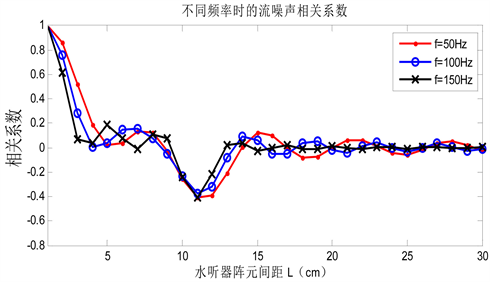Figure 9. Correlation coefficients of flow noise at different frequencies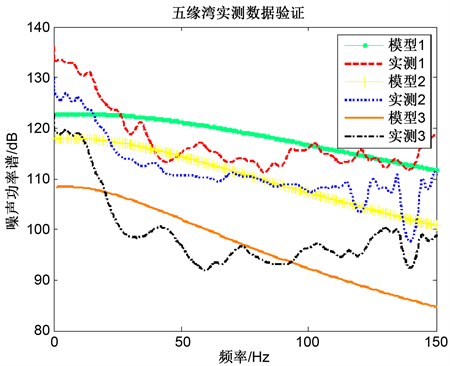Figure 10. Comparison between measured data and model results in wuyuan bay

5. 总结与展望

1) 由于随着拖曳速度的增加，流噪声的谱级明显增加。因此在航行器航行的过程中，应在保持拖曳线列阵水平行进的前提下尽可能的降低拖曳速度。

2) 从水听器自身的尺度出发，可以适当的减小水听器的半径和增加水听器的长度，即可一定程度上可以抑制流噪声。

3) 在对水听器进行布放和封装时，要尽量保证水听器的轴线与护套轴线相重合，这样可以通过使得流噪声径向振速分量相互抵消而达到抑制流噪声的目的。

4) 采用水听器阵列组可以有效抑制流噪声。

NOTES

*通讯作者。

  Corcos, G.M. (1964) The Structure of the Turbulent Pressure Field in Boundary Layer Flows. Journal of Fluid Mechanics, 18, 353-378. https://doi.org/10.1017/S002211206400026X  Carpenter, A.L. and Kewley, D.J. (1983) Investigation of Low Wave Number Turbulent Boundary Layer Pressure Fluctuations on Long Flexible Cylinders. 8th Australasian Fluid Mechanics, 28 Novem-ber-2 December 1983, 9A.1-9A.4.  Liu, Y.W. and Shang, D.J. (2015) The Investigation on Calculating and Measuring the Flow Noise from Underwater Jets. MTS/IEEE Washington, Washington DC, 19-22 October 2015, 1-4.  Zou, N. and Goh, I.N. (2011) Acoustic Vector-Sensor Beam Forming in the Presence of Flow Noise. IEEE International Conference on Acoustics, Speech and Signal Processing, Prague, 22-27 May 2011, 2652-2655. https://doi.org/10.1109/ICASSP.2011.5947030  徐雅倩, 郭高峰, 刘方正. 拖线阵声呐探测技术研究现状及发展趋势[J]. 价值工程, 2017(9): 237-241.  王春旭, 吴崇建, 陈乐佳, 等. 流致噪声机理及预报方法研究综述[J]. 中国舰船研究, 2016, 11(1): 57-71.  Linderman, O.A. (1981) Influence of Material Properties on Low Wavenumber Turbulent Boundary Layer Noise in Towed Array. U.S. Navy Journal of Underwater Acoustics, 31.  时胜国, 于树华, 时洁, 等. 矢量拖线阵不同形状水听器流噪声响应特性[J]. 声学学报, 2016, 41(3): 380-389.  Tao, Y. and Su, H.T. (2014) A Sponge Cover Approach to Reducing Flow Noise of Hydrophone. OCEANS, Taipei, 7-10 April 2014, 1-4. https://doi.org/10.1109/OCEANS-TAIPEI.2014.6964487  应晓伟. 水下突体和声呐导流罩的降噪设计研究[J]. 声学与电子工程, 2014(3): 19-22.  Song, H., He, Y.N., et al. (2016) A Numerical Simulation of Flow-Induced Noise from Cavity Based on LES and Lighthill Acoustic Theory. IEEE/OES China Ocean Acoustics, Harbin, 9-11 January 2016, 1-8. https://doi.org/10.1109/COA.2016.7535723  Arakeri, V.H., Satyanarayana, S.G. and Mani, K. (1991) Studies on Scaling of Flow Noise Received at the Stagnation Point of an Axisymmetric Body. Sound and Vibration, 146, 449-462. https://doi.org/10.1016/0022-460X(91)90701-K  Lauch, G.C. (1992) Flow Noise Scalingat the Stagnation Point of an Ax-isymmetric Body. Sound and Vibration, 154, 568-572. https://doi.org/10.1016/0022-460X(92)90788-Y  Zhang, Y.O., Zhang, T. and Ouyang, H. (2014) Prediction Model of Flow Duct Constriction Noise. Applied Acoustics, 82, 45-52. https://doi.org/10.1016/j.apacoust.2014.03.001